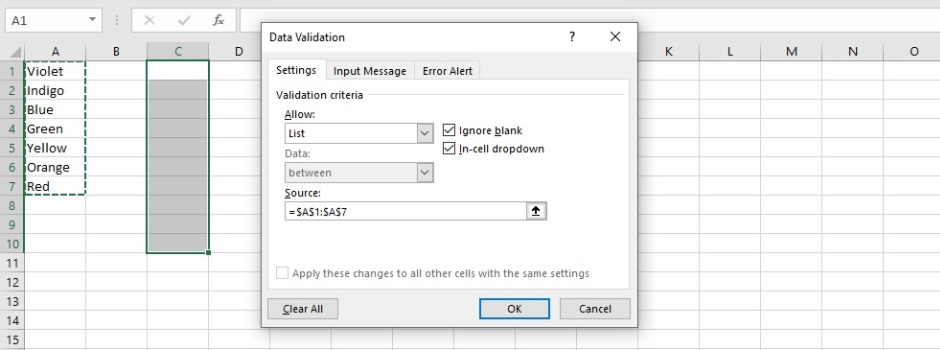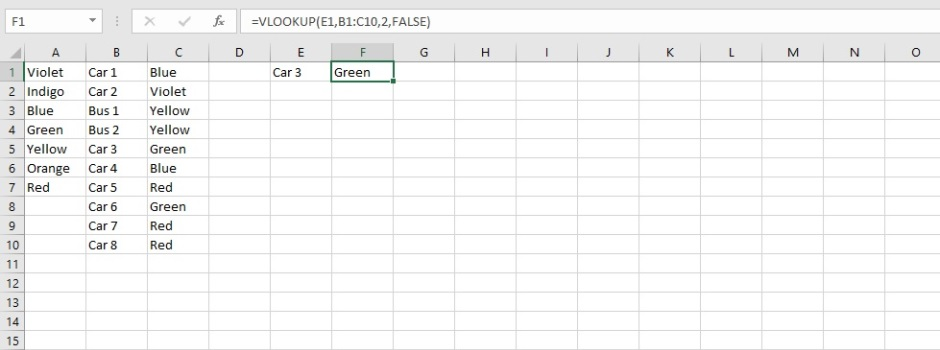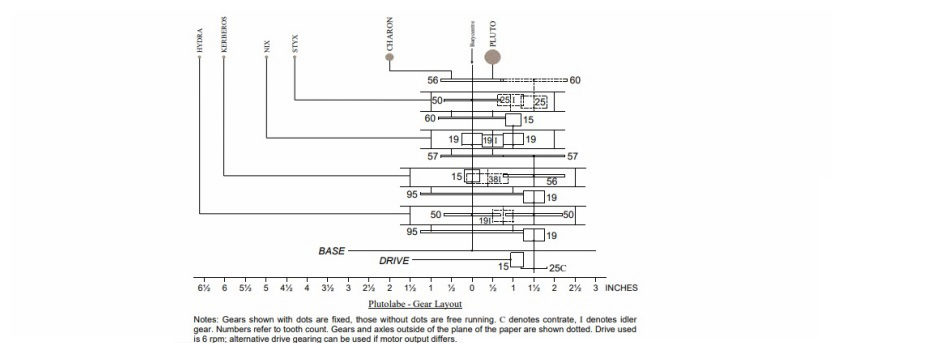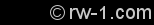﻿ Robin's Website

Robin’s Excel Page

If somebody asked the author of this website if they were allowed one computer with only two applications installed, and no more, then which two they would be? The answer would be simple: A web browser and a copy of Microsoft Excel. Whilst familiar with all of the other Microsoft Office applications, a reasonable knowledge (from previous work) of Adobe programs, such as InDesign, Photoshop and Fireworks, and countless other applications, nothing surely beats Excel for versatility.Above: Excel launcher icons throughout the years. How far back can you remember? The first versions of Excel came out in 1985 (Mac) and 1987 (Windows).

The purpose of this webpage is not to provide a comprehensive tutorial in Microsoft Excel, rather than to maintain a personal record of some of the tools and tricks the author has found out whilst using Microsoft Excel throughout the years; thus, this webpage is not intended to “reinvent the wheel”. There follows no “how-to” instructions, rather just a selection of task-specific tips which the author found useful along the way.

Creating a Dropdown List

Starting with some basic features, the dropdown list is useful with ensuring data input is consistent with a choice of entries and helps with eliminating typing errors where required input can be pre-defined.In this example, cells in column C are highlighted, under “Data”, “Data Validation” is selected, then under “Allow:”, “List” is selected, and the Source is selected as cells A1 to A7. Then a custom error alert can be set to stop entry other than one of the listed colours, with a message alerting the user, if their cell input does not match one of them.

VLOOKUP (& Some Other Basic Formulae)

An extremely useful tool in retrieving data from a table is the VLOOKUP formula. Building on the previous example, the image below lists some vehicles in column B and their colour, as selected from a dropdown list, in column C:In E1, the name of a vehicle listed in column B is entered. In cell F1, the following formula is entered:

=VLOOKUP(E1,B1:C10,2,FALSE)

Where E1 is the value to look up, B1:C10 is the range to search, and 2 = the column number of the range to return the value from. The parameter of FALSE means that VLOOKUP is looking for an exact match for the value (TRUE would mean that an “approximate” match would be used with respect to returning a data value). And so here, because E1 contains the text “Car 3”, cell F1 has looked in the appropriate cells and returned its corresponding colour, Green.

Note 1: If, for example, Car Number or Bus Number ha been entered twice in column B, then the VLOOKUP formula would return the first value it finds, as it searches down the list. For this reason, I would set up a table where the vehicle and its number are initially entered, and have a separate list, where duplicate entries are eliminated, next to which the colour would be entered. This can be achieved using the “COUNTIF” function.

Note 2: If there is no entry for colour, then the value zero might be returned. This issue can be eliminated by embedding the VLOOKUP formula into an IF statement, whereby if the VLOOKUP value zero is returned, “Not Determined” is displayed. Also, when using VLOOKUP formulae, sometimes there may be errors returned in the cell, in which case, I “tidy up” the spreadsheet by embedding the VLOOKUP formula into an ISERROR statement in addition, or instead (ISERROR checks if the returning value is an error or not).

Note 3: Once a spreadsheet is created, it is always useful to lock all cells with a password, other than those where data is to be entered, to help protect those all-important formulae!

Examples where I have used a combination of VLOOKUP, IF, COUNTIF and ISERROR formulae, as well as others, such as “NOW”, which returns the current date and time, include creating Laboratory Information Management (LIMS) systems, where data generated is maintained and accessed in a database (Microsoft Excel provides a more versatile means of performing numerical calculations on data than database programs, such as Microsoft Access), maintaining inventories of items which have an expiry date, keeping staff holiday records and ensuring there are no clashes with other members of staff trained in the same procedures who have already booked time off, and maintaining medication stocks of clients/reordering forms within a residential care home setting. These are just a few examples and, of course, the number of possible applications using these formulae is seemingly endless.

Rounding Numbers

Rounding numbers using the formula =ROUND(number, num_digits) is important if applying IF formulas to compare a value against a specification. For example, if a value is always reported to one decimal place, there is no use in comparing a value of 8.03 with a specification of 8.0; the IF formula will state that 8.03 is greater than 8.0. However, if the 8.03 is rounded to 8.0, it will not be greater than the specification (in any circumstances where rounding up is always necessary, the ROUND function can be replaced by the ROUNDUP function).

Reporting to Significant Figures

In some situations, values may be reported to a number of significant figures, as opposed to a number of decimal places. Values may be reported this way as per the following example:

=ROUND(A1,3-(1+INT(LOG10(ABS(A1)))))

Where the value in cell A1 is to be reported to (as an example) 3 significant figures. (3 can be replaced by another value or by a cell reference, if the number of significant figures to report to varies)

Returning the Sheet Name in a Cell

This can be achieved using the following formula (in a workbook that has already been saved somewhere):

=MID(CELL("filename",A1),FIND("]",CELL("filename",A1))+1,255)

Presenting Tables of Read-Only Data on a Webpage

This is another use I have found for Excel. A workbook containing information (only) in tables in Excel is saved as a webpage. The first worksheet is then added to a webpage using an iframe in HTML and the other worksheets can be viewed in the webpage as displayed in a browser, by clicking on the different worksheet tabs, as one would do in Excel itself.

Protecting / Unprotecting all Worksheets

For protecting all worksheets in a workbook, run the following in Visual Basic for Applications (VBA):

Sub ProtectAllWorksheets()

Dim ws As Worksheet

Dim Pwd As String

For Each ws In ActiveWorkbook.Worksheets

Next ws

End Sub

For unprotecting multiple worksheets in a workbook, run the following in Visual Basic for Applications (VBA):

Sub UnProtectAllWorksheets ()

Dim ws As Worksheet

Dim Pwd As String

Pwd = InputBox("Enter the password to unprotect all worksheets", "Unprotect Worksheets")

On Error Resume Next

For Each ws In Worksheets

Next ws

If Err <> 0 Then

MsgBox "Incorrect password entered. All worksheets could not " & _

End If

On Error GoTo 0

End Sub

Using Excel for Drawing

For all of its power in handling numerical data, Excel can be surprisingly useful when it comes to drawing. Not only for flow-charts and the like, but as seen here, it is a useful tool for scaled drawings. The use here, came in the form of creating diagrams of Orreries (models showing the rotation of planets around a star, or moons around a planet) which were constructed out of Meccano. An example is shown here:Prior to creating the drawings, which involved using cell borders for the horizontal and vertical lines, for this purpose it was necessary to make the cells on the worksheets square. This can be achieved by selecting “Page Layout” under the “View” tab. Column widths and row heights will now be in ruler units (inches, centimetres or millimetres, depending on how your version of Excel is set up). Then, adjust the row heights and column widths to an identical value as seen fit. By changing the view setting from “Page Layout” back to “Normal”, row heights and column widths will be displayed in points again.

Note: To change the ruler units visible in Page Layout (e.g. from mm to inches or vice versa), then under “File”, select “Options”, then “Advanced” and under the heading “Display”, change the Ruler units using the dropdown menu and click on “OK” to apply.

How to Get Directory/Folder Names and File Names from File Manager/Windows Explorer into Excel

In a situation where there are a large quantity of files and folders stored on a computer and the names of them all need to be recorded, I have written a guide on how to do this (in Windows). Note – for the filenames, see Note 2 on page 4. The guide can be viewed on the link Here.